Derivation of Taylor Series Expansion

Objective:

Given f(x), we want a power series expansion of this function with respect to a chosen point xo, as follows: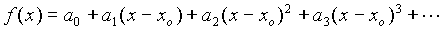(1)
( Translation: find the values of a0, a1,  of this infinite series so that the equation holds. )

Method:

The general idea will be to process both sides of this equation and choose values of x so that only one unknown appears each time.

To obtain ao: Choose x=xo in equation (1). This results in

a0 = f(xo)

To obtain a1: First take the derivative of equation (1)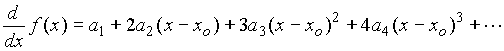(2)
Now choose x=xo.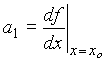To obtain a2: First take the derivative of equation (2)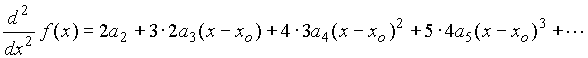(3)

Now choose x=xo.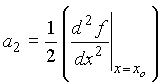To obtain a3: First take the derivative of equation (3)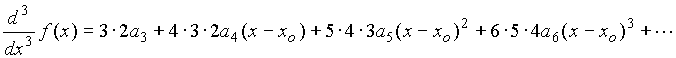(4)

Now choose x=xo.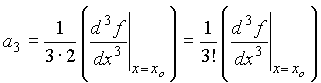To obtain ak: First take the kth derivative of equation (1) and then choose x=xo.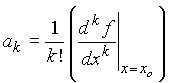Summary:

The taylor series expansion of f(x) with respect to xo is given by: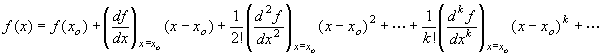Generalization to multivariable function:

Let x, y and z be the three independent variables,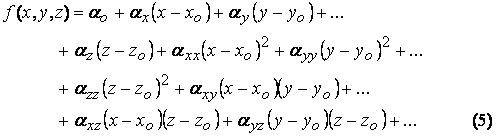Using similar method as described above, using partial derivatives this time,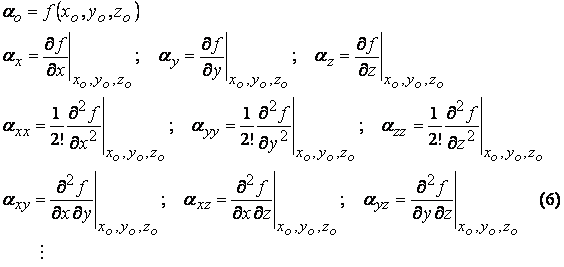For the general case of  n  independent variables,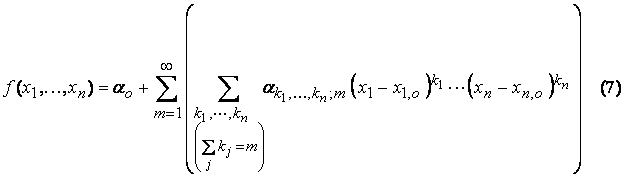where the coefficients are given by,(Note: the procedure above does not guarantee that the infinite series converges. Please see Jenson and Jeffreys, Mathematical Methods in Chemical Engineering, Academic Press, 1977, for a thorough discussion on how to analyze the convergence of the resulting series.)

This page is maintained by Tomas B. Co (tbco@mtu.edu). Last revised 06/10/2007.

Tomas B. Co
Associate Professor
Department of Chemical Engineering
Michigan Technological University
1400 Townsend Avenue
Houghton, MI 49931-1295